Voltage conversions Conversion topics LabHelper topics LabHelper menus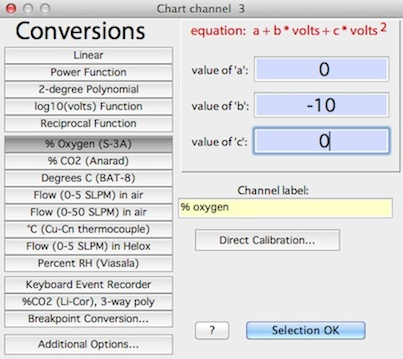This window lets you specify how raw input voltages are converted into appropriate units (such as speed, temperature, flow rate, gas concentration, etc.).  The standard window looks like this (but note that you can produce customized buttons using the custom conversions option in the EDIT menu):

Top of page

Voltage conversions take several forms:

•   Raw input voltages  volts x 1).  NOTE:  oscilloscope channels are used with the raw voltage option only, in order to reduce processing requirements and speed up the sampling rate.

•   Power functions:

value = A + B x (voltsexponent )

If the exponent = 1.0, it is better to use the faster two-order polynomial option (below).

 CAUTION:   if you select a non-integer exponent and LabHelper reads a negative voltage, the resulting operation is mathematically meaningless and the recorded value for that sample is set to zero.  Make sure that a non-integer exponent 'sees' (i.e., is applied to) only positive voltages!

•   two-order polynomial:

value = A + B x volts + C x (volts2)

Polynomials have several advantages over power functions.  They handle negative voltages easily, and compute much faster than power functions.

•   exponential:

value = A + B x e(C x volts)

Exponentials accept negative voltages but do not compute as fast as polynomials.

•   ONE channel can use a three-order polynomial conversion:

value = [ A + BxV + CxV2 + DxV3 ] x E

where V is a scaled input voltage:

Top of page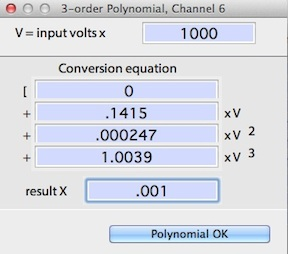The default polynomial scaling is conversion of input volts into millivolts (mV, = volts X 1000).  However, you can enter any appropriate conversion factor for input volts.  This is particularly important if you are using a voltage divider, and you are using an instrument with a pre-defined polynomial conversion factor.  You have to account for the effect of the in order to properly use the polynomial.  Accordingly, the input voltage scaling factor should incorporate the voltage reduction (and any other needed scaling) so as to yield the correct output of the instrument for the polynomial.  In this example, the instrument's output voltage is multiplied by 1,000 to obtain millivolts, and the result is multiplied by .001 to get the correct value.  For most instruments using the three-order polynomial, both the input conversion factor and the result conversion factor are set to 1.0.

• The standard button for this option is labeled "%CO2 (LiCor 6251)", since it was implemented to handle calculation of CO2 concentration from a LiCor 6251 analyzer.  The LiCor came with a factory-defined polynomial conversion based on millivolt output that needs to be scaled (newer versions of this analyzer have built-in linearizing circuits and don't need complex conversions).  However, with appropriate changes to the various coefficients, this routine can be used for most instruments requiring a polynomial conversion.

• Note that a few instruments use a higher-order polynomial for converting recorder output voltages.  Options for these units include after-the-fact conversion (in LabAnalyst or another analysis program) or computing a three-order equivalent of a higher-order polynomial, provided this can be done with sufficient accuracy.

•   Keyboard event recorder setting responds to keyboard input by converting certain keystrokes into numeric values.  The event value always starts at zero.  The event recorder responds to these keys:

• Numbers from 0 to 9 convert to equivalent event values.  Note: pressing these keys places a marker in the file.

• The arrow keys: 'up' and 'right' add one to the current event value; 'down' and 'left' subtract one from the current value.  No marker is entered when arrow keys are struck.

• The 'home' key: converts to an event value of zero; no marker is entered.

Top of page

•   Breakpoint conversions:

A 'breakpoint' conversion is a complex set of calculations in which different equations are applied to input voltages depending on where they lie in relation to a specified 'breakpoint' voltage.  For example, you might apply a linear conversion to all data of <2 volts, but a polynomial conversion for values >= 2 volts.  This window lets you set the breakpoint conversion conditions: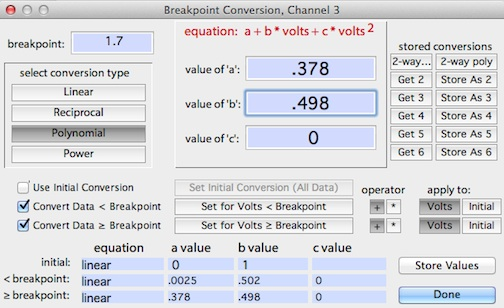Each breakpoint conversion operation involves up to three different equations, each of which can be linear, exponential, 2-order polynomial, or power.   The initial conversion is (optionally) applied to all data regardless of voltage.  The other two 'secondary' conversions are (optionally) applied to data above or below breakpoint, respectively.  The secondary conversion can either be added to or multiplied by the results of the initial conversion (this is selected with the 'operator' buttons) and can use either raw voltages or the values from the initial conversion (selected with the 'apply to' buttons).  Note that the breakpoint itself is always specified as a raw input voltage.

The window shows a summary table of the three equations at the bottom.  You enter the coeffients in the three edit fields at top center, and then click the appropriate button to enter them into the initial or secondary conversions. The 'get x' and 'store as x' buttons let you save and retrieve up to 6 complete breakpoint conversions in memory.  To save them more permanently in a preferences file, click the 'store values' button or use the 'store preferences' option in the FILE menu.  If you save them in a file called "LabHelper prefs" and it is located in the same folder as the LabHelper program, it will be loaded automatically at startup.

Top of page

•   Conversion options: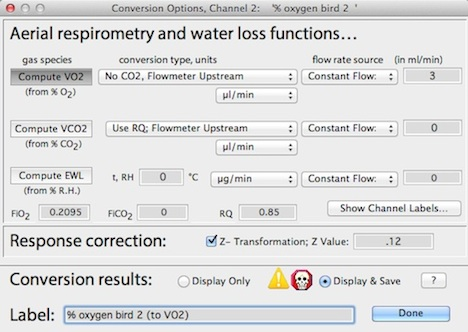This lets you apply secondary conversions to your data; most of these options are specialized for aerial respirometry.  You can compute VO2 VCO2, or VH2O, using several basic conversion algorithms (more comprehensive options for respirometry are available in the LabAnalyst analysis program).  Here is an example showing calcualtion of oxygen consumption, with flow rate obtained from another channel:

This example also shows use of the response correction (Z-transformation).  You have the option of showing conversions during acquisition but saving the raw data only, or of saving the converted data.

•   pre-defined conversions designed for commonly-used instruments can be selected from the labeled buttons.  You can create your own customized buttons and conversion factors using the custom conversions option in the EDIT menu.

•   When using a DataTaker, a button labeled "°C (Cu-Cn thermocouple)" is available (in the default mode).  Clicking this button instructs the DataTaker to return a compensated temperature (in degrees C), instead of voltage.  Note that if another kind of input device is used with this option, erroneous data will be returned.

•   The Direct Calibration button opens a window for keyboard entry of values, which are then compared with measured voltages to produce a conversion equation by linear regression, power function, or 2-order polynomial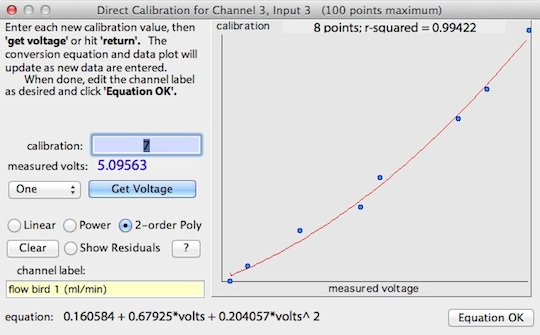The window shows the entered points, the regression line and regression coefficient, and the conversion equation.  At least three points must be entered before a valid conversion is generated.  For each point, sent the instrument in question to a particular value, enter that value in the edit field, and click the 'get voltage' button (or hit return).  Repeat as necessary with different instrument values.  Note that negative inputs (values or voltages) are not accepted when using power functions.  A display of the regression residuals is shown when the residuals button is clicked.  Here is an example (a 2-order polynomial conversion is selected).

•    When done, click the 'Conversion OK' button.

• Note that although entering voltage conversion equations can be tedious, particularly for multi-channel sampling protocols, you can save conversions in 'setup' files for immediate retrieval and re-use.

 go to: